﻿

### A note on embeddings of projective spaces

#### Abstract

Let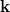$\textbf{k}$ and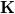$\textbf{K}$ be commutative fields, and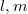$l,m$ integers with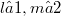$l ≥ 1, m ≥ 2$. Suppose that there exists an embedding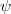$\psi$ of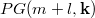$PG(m + l,\textbf{k})$ to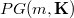$PG(m,\textbf{K})$, then we have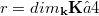$r = dim_{\textbf{k}}\textbf{K} ≥ 4$ and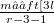$m ≥ ≤ft [{3l}\over{r-3}\right] - 1$. Conversely, there exists an embedding$\psi$ of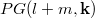$PG(l + m,\textbf{k})$ to$PG(m,\textbf{K})$ if$m ≥ ≤ft [{3l}\over{r-3}\right] - 1$ and if (1)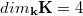$dim_{\textbf{k}}\textbf{K} = 4$, or (2)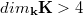$dim_{\textbf{k}}\textbf{K} > 4$ and$\textbf{K}$ is a cyclic extension of$\textbf{k}$ with some additional conditions on l and r.

DOI Code: 10.1285/i15900932v19n2p285

Full Text: PDF